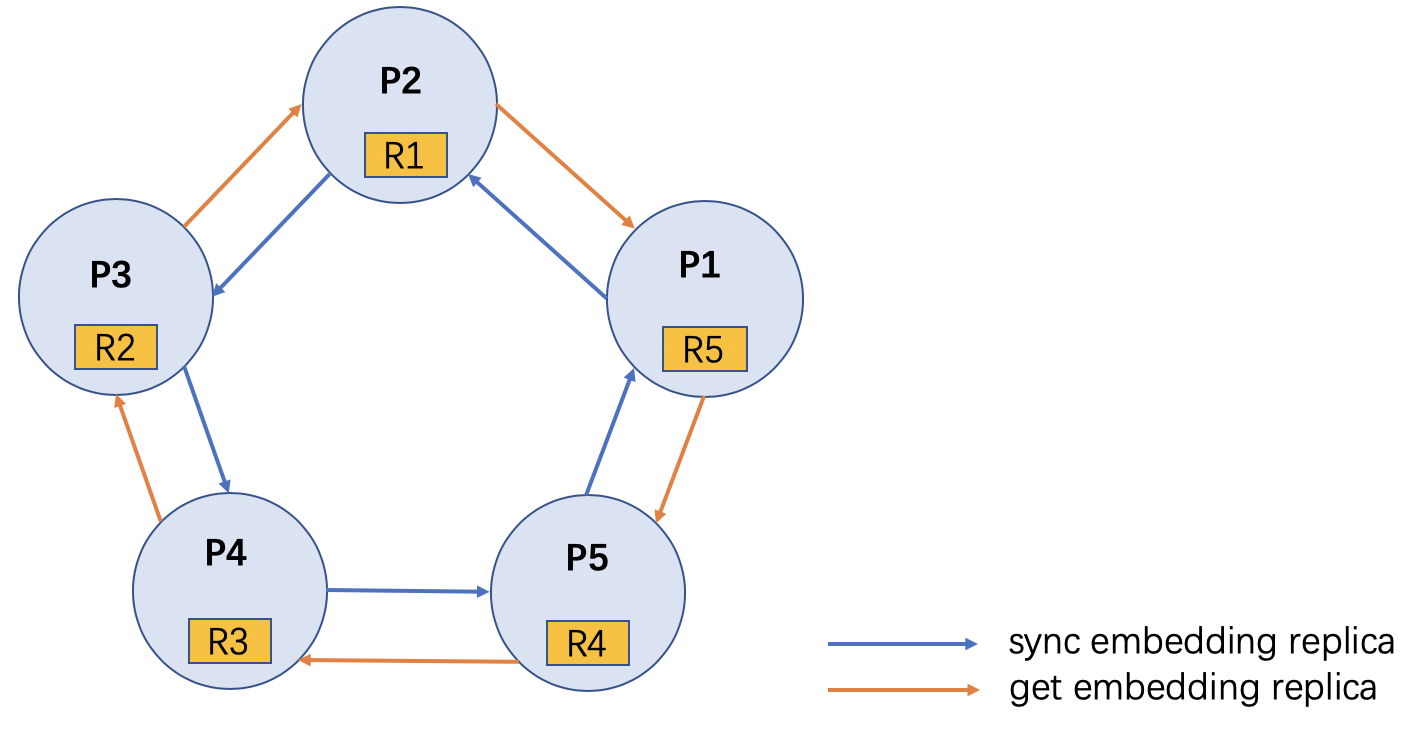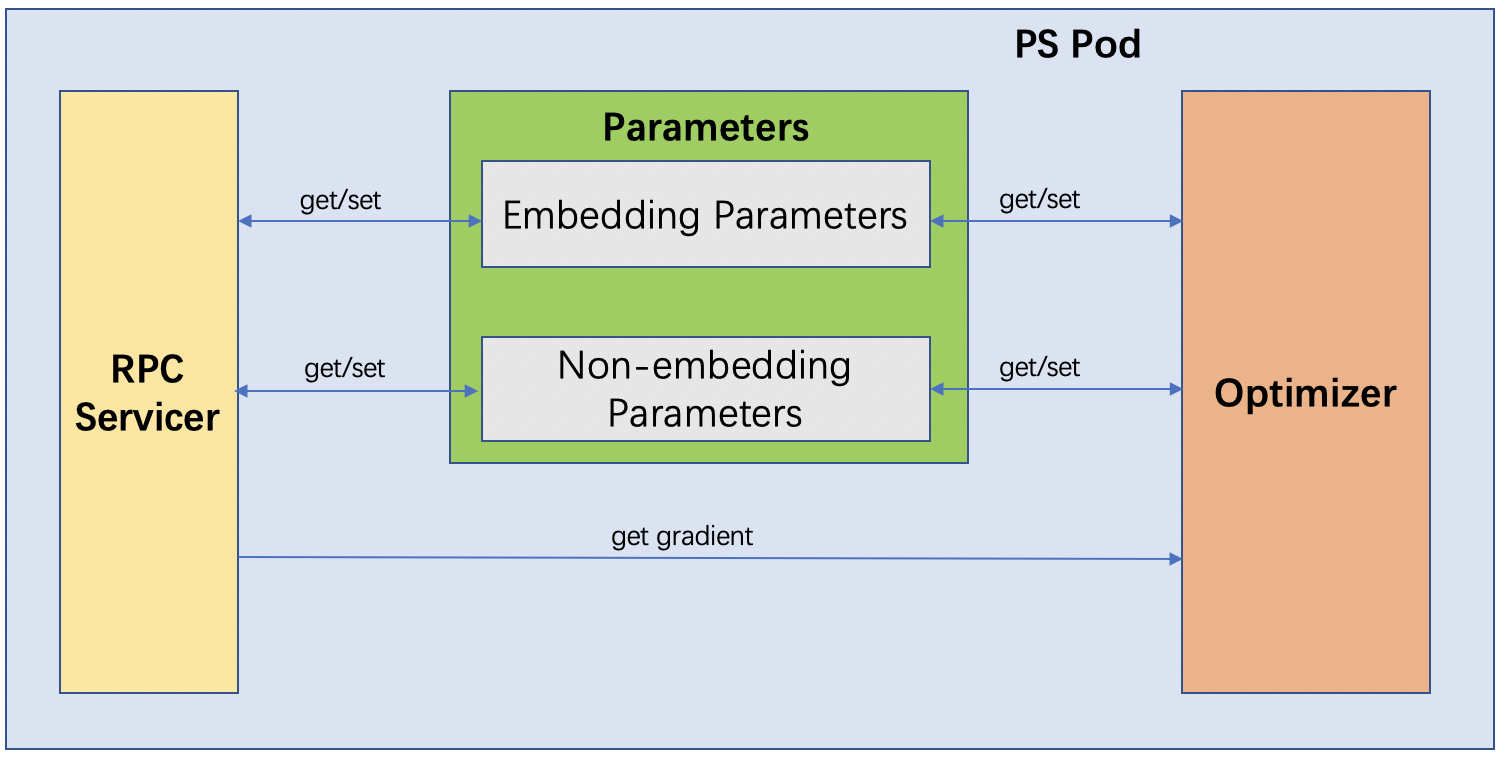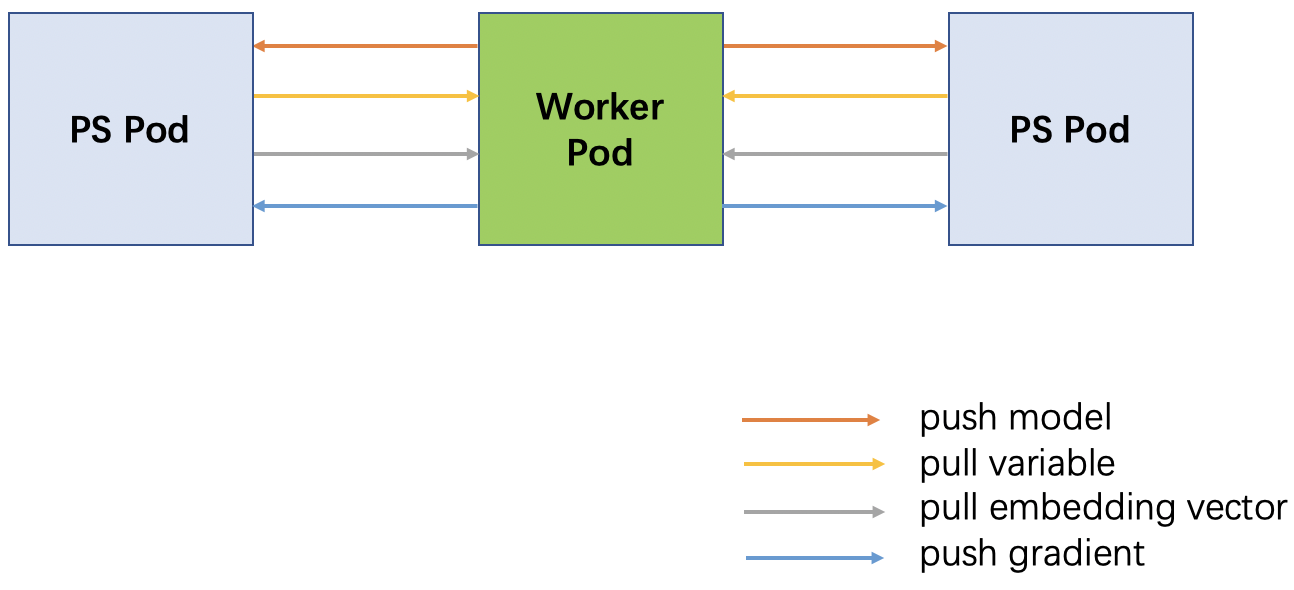# ElasticDL Parameter Server Design

This document describes the design of a distributed parameter server for ElasticDL.

## Motivation

Parameter server (PS) stores model parameters which are used by workers. Workers get model parameters from PS, compute gradients using different training data and send computed gradients to PS. PS iteratively updates these model parameters using gradients sent by workers. A PS based distributed training system can use an arbitrary number of workers to support the scalability of training data size.

We want to have one or more PS instances in each ElasticDL job. One reason is that models could be large and overrun the memory space of a single PS instance. In such case, we need to partition the model and store different partitions in different PS instances. Even if the model is not too big and fits in the memory of a single PS instance, we might still want to partition the model, as this distributes the model parameter communication from workers among PS instances. This also distributes the computation on PS such as parameter optimization.

ElasticDL is a Kubernetes-native fault-tolerable deep learning system. An ElasticDL distributed PS consists of multiple PS pods, with each PS pod as a PS instance. A failed PS pod will interrupt the training. We can relaunch any failed PS pod and recover the corresponding model parameter partition to support PS fault tolerance.

In the following sections, we will explain the design of the ElasticDL distributed PS with fault-tolerance in detail, including how to partition the model, store model parameters, access parameters from workers, initialize parameters, update parameters from gradients and support PS fault-tolerance.

## Model Parameter Partition

For a distributed PS with N PS pods, each PS pod stores a model parameter partition. It is noticeable that Kubernetes may preempt some PS pods. In such a case, N might not be a constant. However, we can overcome this case by setting PS pods having higher priority than worker pods in a job. In case a PS pod is preempted, the master will relaunch it using Kubernetes APIs. Since PS pods have a higher priority than worker pods, if there are still some worker pods running, the relaunch will succeed by using either idle or preempted Kubernetes resources. If no worker pods left, ElasticDL has to wait for Kubernetes resources to continue the training. Thus, we can assume that N is a constant number in ElasticDL.

We consider two kinds of model parameters:

1. non-embedding parameters, and
2. embedding tables

Theoretically, non-embedding parameters might have tremendous size and require partitioning. However, in practice, limited by the amount of GPU memory, researchers don’t often define models depending on huge dense tensor parameters. Hence in this design, we don’t partition non-embedding parameters; instead, we place each of them on a PS pod.

For a non-embedding parameter, we select its PS pod PSᵢ using a hashing function hash and the parameter name p_name:

``````i = hash(p_name) % N
``````

Each embedding layer has an embedding table which maps a discrete ID i to an embedding vector vᵢ. Embedding tables could be huge, especially in some recommending and ranking models. Thus, we partition each embedding table and store every partition in an unique PS pod. For an embedding vector vᵢ, we select the (i mod N)-th parameter server to store it.

## Model Parameter Storage

Each PS node has a dictionary-based data structure to store its partition of model parameters.

We choose to store each non-embedding parameter using the parameter name as its key, and a `tf.Variable` instance as its value. This is because that we want to update non-embedding parameters directly by TensorFlow optimizers.

If a model has one or more embedding layers, a minibatch of training data contains a set of discrete IDs. These discrete IDs correspond to a set of embedding vectors. The worker needs to pull these embedding vectors from their corresponding PS pods using the embedding layer name and the discrete IDs. To store an embedding vector, We use its corresponding embedding layer name and discrete ID as the key, and a 1-D numpy.ndarry as the value.

## Model Parameter Access from Worker

Each PS pod has a RPC servicer to provide RPC services. Workers use RPC services to pull model parameters. `pull_variable` service is to pull all non-embedding parameters. `pull_embedding_vector` service is to pull embedding vectors specified by an embedding layer name and a list of discrete IDs.

``````service PServer{
rpc pull_variable(PullModelRequest) returns (PullModelResponse);
rpc pull_embedding_vector(PullEmbeddingVectorRequest) returns (Tensor);
}
``````

## Model Parameter Initialization

We use lazy initialization for model parameters in PS. PS does not have the model definition. Even if PS has the model definition, it cannot initialize Keras subclass model parameters, as only a forward-pass with a minibatch of data can initialize the parameters. Thus workers are responsible for initializing parameters and push the initialized parameters to corresponding PS pods.

Each PS pod has a parameter initialization status, which is `False` after the PS pod launch. When a worker tries to get non-embedding parameters from the PS pod through a RPC call `pull_variable`, the PS pod tells the worker that the parameter initialization status is `False` in response. If the worker has already initialized non-embedding parameters, it sends non-embedding parameter values to the PS pod by a gRPC call `push_model`. `push_model` is a RPC service in the PS pod.

``````service PServer{
rpc push_model(Model) returns (google.protobuf.Empty);
}
``````

If worker has not initialized non-embedding parameters, since the worker has the model definition and some training data, it can run a forward-pass to initialize them first before `push_model`.

When the PS pod receives non-embedding parameters in its first RPC service for `push_model`, it initializes non-embedding parameters and sets the parameter initialization status as `True`.

For an embedding vector, the corresponding PS pod will initialize it in the first `pull_embedding_vector` service that contains this embedding vector. The PS pod needs the embedding vector size and the initialization method for the initialization. The embedding vector size and the initialization method are in the model definition and workers can send them in `push_model` to PS pods together with non-embedding parameter values.

Thus, we introduce two data structures: `Parameters` and `EmbeddingTable`. `Parameters` stores both embedding parameters and non-embedding parameters. `EmbeddingTable` stores embedding vectors, which is a subset of an embedding table.

``````class Parameters(object):
def __init__(self):
# Parameter initialization status
self.parameter_init_status = False
# Non-embedding parameter dict, maps parameter name to tf.Variable instance
self.non_embedding_params = {}
# Embedding table dict, maps embedding layer name to `EmbeddingTable` instance
self.embedding_params = {}

class EmbeddingTable(object):
def __init__(self, dim, initializer):
# Embedding vector dict, maps ID to 1-D numpy.ndarray
self._embedding_vectors = {}
# the dimension of embedding vectors
self._dim = dim
# the initializer name for initializing embedding vectors
self._initializer = initializer
``````

## Model Parameter Update

A worker computes gradients in each training iteration, which contain gradients for non-embedding parameters and some embedding vectors if applicable. The worker partitions these gradients using their corresponding parameter names or discrete IDs for embedding vectors. Then the worker sends gradient partitions to their corresponding PS pods by RPC calls `push_gradient`.

``````service PServer{
}
``````

When a PS pod receives gradients in `push_gradient`, it uses a TensorFlow optimizer to apply gradients to non-embedding parameters.

We have already implemented an `OptimizeWrapper` to sparsely update embedding vectors. `OptimizeWrapper` uses corresponding embedding vectors to form a temporary variable, applies gradients to this temporary variable, and writes results back to these embedding vectors. The PS pod can use this OptimizeWrapper directly to update embedding vectors.

In asynchronous SGD, the PS pod can apply gradients directly to model parameters once it receives gradients. For synchronous SGD, the PS pod accumulates `grads_to_wait` gradients from workers then updates model parameters with these gradients. `grads_to_wait` is an ElasticDL argument specified by the user.

## PS Fault Tolerance

When the master detects that a PS pod fails, it will relaunch it using Kurbernetes APIs to keep the number of PS pods N constant. After the relaunch, the PS pod recovers its partition of model parameters so that ElasticDL can continue the training job.

### Fixed Domain name for PS Pod

Each PS pod provides RPC services for workers. Workers are using RPC stubs to send RPC service requests to PS pods. RPC stubs require PS pod domains. Because ElasticDL is Kubernetes-native, the master can use Kubernetes services to launch/relaunch PS pods with fixed domain names. The master sends these domain names to workers as arguments when launching worker pods. In such way, workers do not need to re-configure RPC stubs after a PS pod relaunch.

### Model Parameter Recovery

The model may contain one or more embedding layers with embedding tables as their parameters. If so, a minibatch of training data in a worker contains some embedding IDs, which correspond to a subset of embedding tables. The worker pulls all non-embedding parameters and only a subset of embedding tables from PS pods in the training. Thus, the PS pod can recover non-embedding parameters from workers but not embedding tables.

For non-embedding parameters, the PS pod can recover them from workers in the same way as the parameter initialization by setting its parameter initialization status as `False`.

For embedding tables, PS creates replicas to support fault-tolerance. For each PS pod PSᵢ, it can store M replicas of its embedding table partitions in M PS pods indexed from (i+1) mod N to (i+M) mod N. The relaunched PS pod can recover embedding tables from one of its replicas.

### Embedding Replica

Assume Eᵢ is the embedding table partition in PS pod PSᵢ, it has M replicas stored in PS pods indexed from (i + 1) % N to (i + M) % N. Also, PSᵢ stores M other PS pod replicas covering Eᵢs where i ranges from (i - M) mod N to (i - 1) mod N.

PSᵢ maintains M updated embedding vector key sets UKSᵢ(j) for j ∈ [0， M). When PSᵢ sparsely updates its embedding table partition Eᵢ, it also adds the updated embedding vector keys into these M sets.

PSᵢ also periodically synchronize the replicas stored in it from PS pods indexed from (i - M) mod N to (i - 1) mod N. The synchronization frequency can be several seconds.

PSᵢ uses M RPC calls `SynchronizeEmbedding` the replicas store in it. `replica_index` values in `SynchronizeEmbeddingRequest` are from (i - M) % N to (i - 1) % N.

When PSᵢ needs to recover its embedding vectors after relaunch, it chooses a pod PSⱼ from indexed from (i + 1) mod N to (i + M) mod N which is still alive. PSᵢ uses a RPC call `GetReplica` to get its replica from PSⱼ.

Following diagram shows the RPC calls among PS pods for PS fault-tolerance:Here, we set up 5 PS pods, and set embedding replica number M to 1. PS pod 2 has an embedding replica `R1` of PS pod 1. It will periodically synchronize the replica from PS pod 1. If PS pod 1 is dead, the master will relaunched it and it needs to get the replica from PS pod 2 after relaunch.

``````message SynchronizeEmbeddingRequest {
int32 replica_index = 1;
}

message SynchronizeEmbeddingResponse {
repeated Tensor embedding_vectors = 1;
}

service PServer{
# RPC service for replica synchronization
rpc SynchronizeEmbedding(SynchronizeEmbeddingRequest) returns (SynchronizeEmbeddingResponse);

# RPC service for PS to recover embedding vectors after relaunch
rpc GetReplica(SynchronizeEmbeddingRequest) returns (SynchronizeEmbeddingResponse);
}
``````

Each PS pod has a thread dedicated to the replica synchronization:

``````# T is the number of seconds for synchronization frequency
# Assume current PS is PS(i), self._stub[index] is the stub for PS((i - index) % N)'s gRPC server.
# self.replicas[index] is the replica for PS((i - index) % N).

req = elasticdl_pb2.SynchronizeEmbeddingRequest()
while still training:
time.sleep(T)
for index in range(M):
req.replica_index = index
updated_vectors = self._stub[replica_index].SynchronizeEmbedding(req)
update self.replicas[index] from updated_vectors.embedding_vectors
``````

## Diagram

Following diagram shows the details inside a PS pod:Following diagram shows the RPC calls between a worker pod and two PS pods:Please note that there are many worker pods in an ElasticDL job, and each worker pod will have RPC connections with all the PS pods. Here we only show one worker pod for simplicity.

## Appendix

### Message Definition

``````message Tensor {
enum DataType {
BOOL = 0;
INT16 = 1;
INT32 = 2;
INT64 = 3;
FP16 = 4;
FP32 = 5;
FP64 = 6;
}
string name = 1;
DataType data_type = 2;
repeated int64 dim = 3;
bytes content = 4;
repeated int64 indices = 5;
}

message EmbeddingTableInfo{
string name = 1;
repeated int64 dim = 2;
string initializer = 3;
}

message Model {
int64 version = 1;
repeated Tensor variables = 2;
repeated EmbeddingTableInfo embedding_table_info = 3;
}

message PullVariableRequest{
int64 version = 1;
}

message PullVariableResponse{
bool model_init_status = 1;
Model model = 2;
}

int32 model_version = 1;
repeated Tensor gradients = 2;
}

bool accepted = 1;
int32 model_version = 2;
}

message PullEmbeddingVectorRequest{
string name = 1;
repeated int64 ids = 2;
}

message SynchronizeEmbeddingRequest {
int32 replica_index = 1;
}

message SynchronizeEmbeddingResponse {
repeated Tensor embedding_vectors = 1;
}
``````

### RPC Definition

``````service PServer{
# pull trainable tensorflow variables created by Keras layers
rpc pull_variable(PullVariableRequest) returns (PullVariableResponse);

# pull embedding vectors in ElasticDL embedding layers
# Do we need to create a new message `PullEmbeddingVectorRequest` rather than use `Tensor`?
rpc pull_embedding_vector(PullEmbeddingVectorRequest) returns (Tensor);

# push trainable tensorflow variables and meta info for ElasticDL embedding layers
rpc push_model(Model) returns (google.protobuf.Empty);

# PS to recover embedding vectors after relaunch
rpc get_replica(SynchronizeEmbeddingRequest) returns (SynchronizeEmbeddingResponse);

# PS replica synchronization
rpc synchronize_embedding(SynchronizeEmbeddingRequest) returns (SynchronizeEmbeddingResponse);
}
``````

### Data Structure

``````class Tensor(object):
def __init__(self, name=None, value=None, indices=None):
self.name = name
self.value = value
self.indices = indices

@classmethod
def from_tensor_pb(cls, tensor_pb):
"""Create an ElasticDL Tensor object from tensor protocol buffer.

Return the created tensor object.
"""
pass

def to_tensor_pb(self):
pass

def to_tf_tensor(self):
pass

def to_ndarray(self):
pass

def serialize_to_pb(tensor, pb):
"""Serialize ElasticDL Tensor to tensor protocol buffer."""
pass

def deserialize_from_pb(pb, tensor):
"""Deserialize tensor protocol buffer to ElasticDL Tensor."""
pass

def tensor_pb_to_ndarray(tensor):
"""Deserialize tensor protocol buffer and return a numpy ndarray."""
pass

def tensor_pb_to_tf_tensor(tensor):
"""Deserialize tensor protocol buffer and return a TensorFlow tensor."""
pass
``````
``````# In `Parameters`, interfaces `set_*_param` have two arguments, `value` and `name` (or `layer_name`).

# If `value` is a ElasticDL `Tensor` instance, `name` can be None.

# Otherwise `value` is a numpy ndarray, and `name` must be specified.

class Parameters(object):
def __init__(self):
# Parameter initialization status
self.parameter_init_status = False
# Non-embedding parameter dict, maps parameter name to tf.Variable instance
self.non_embedding_params = {}
# Embedding table dict, maps embedding layer name to `EmbeddingTable` instance
self.embedding_params = {}

@property
def non_embedding_params(self):
return self._non_embedding_params

def set_embedding_param(self, value, layer_name=None):
pass

def get_embedding_param(self, layer_name, ids):
return self._embedding_params.get(layer_name).get(ids)

def set_non_embedding_param(self, value, name=None):
pass

def init_non_embedding_param(self, value, name=None):
pass

def set_meta_info(self, layer_name, dim, initializer):
pass

class EmbeddingTable(object):
def __init__(self, dim, initializer):
# Embedding vector dict, maps ID to 1-D numpy.ndarray
self._embedding_vectors = {}
# the dimension of embedding vectors
self._dim = dim
# the initializer name for initializing embedding vectors
self._initializer = initializer

def get(self, ids):
values = []
for id in ids:
if id not self._embedding_vectors:
val = initialize_embedding_vector(self._dim, self._initializer)
else:
val = self._embedding_vectors.get(id)
values.append(val)
return np.concatenate(values).reshape(len(ids), -1)

def set(self, ids, values):
pass
``````

### Some pseudocodes

Here is the pseudocode for a worker to pull variable from the PS. If the non-embedding variables are not initialized, the PS will tell the worker to initialize them and report to the PS.

``````class PServer(elasticdl_pb2_grpc.PServerServicer):
...
def pull_variable(self, request):
res = PullModelResponse()
if self._need_initialize_model:
res.model_init_status = True
return res
res.model_init_status = False
res.model = self._get_model() # get model in this PS instance
return res

def push_model(self, request):
model = request.model
... # initialize model in this PS instance

class Worker(object):
...
def pull_variable(self):
# for-loop should be implemented in multithread
for ps_index in range(self._ps_node_num):
req = PullModelRequest() # create request code keeps the same with current code
res = self._stub[ps_index].pull_variable() # pull variable from PS
if res.model_init_status:
# worker initializes its model here if needed
model = serialize_model_to_pb()
self._stub[ps_index].push_model(model) # get model in this worker
req = PullModelRequest() # create request code keeps the same with current code
res = self._stub[ps_index].pull_variable() # pull variable from PS
if res.model_init_status:
raise Error or try a pre-defined constant times
``````

Here is the pseudocode for getting replica from specified PS pod and synching replicas:

``````# T is the number of seconds for synchronization frequency

# Assume current PS is PS(i), self._stub[index] is the stub for PS((i - index) % N)'s gRPC server.

# self.replicas[index] is the replica for PS((i - index) % N).

req = elasticdl_pb2.SynchronizeEmbeddingRequest()
while still training:
time.sleep(T)
for index in range(M):
req.replica_index = index
updated_vectors = self._stub[replica_index].SynchronizeEmbedding(req)
update self.replicas[index] from updated_vectors.embedding_vectors

def SynchronizeEmbedding(self, request, _):
synch_embeddings = elasticdl_pb2.SynchronizeEmbeddingResponse()
# self.UKS are the M updated embedding vector key sets in current PS
# self.embedding_vector are the embedding vectors in current PS
with self.lock():
assign synch_embeddings.embedding_vectors from self.embedding_vector
self.UKS.clear()
return synch_embeddings

def GetReplica(self, request, _):
replica = elasticdl_pb2.SynchronizeEmbeddingResponse()
assign replica.embedding_vectors from self.replicas[request.replica_index]
return replica
``````